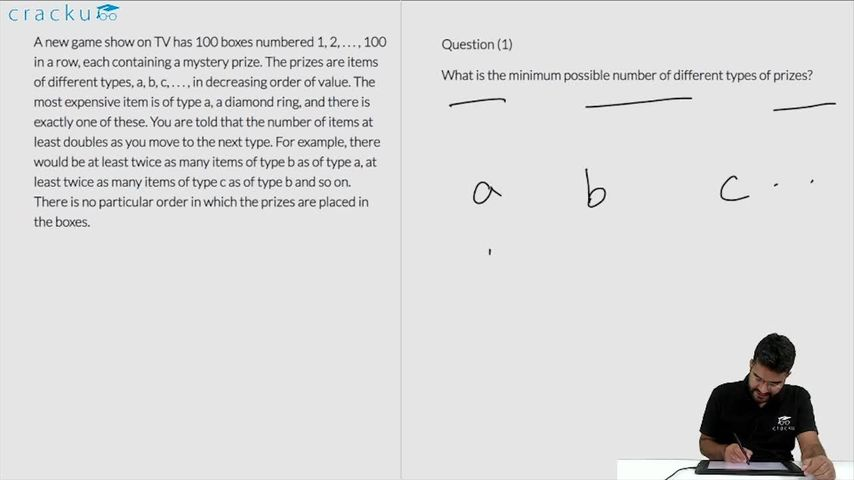### CAT 2019 Question Paper (Slot 1) Question 63

Instructions

A new game show on TV has 100 boxes numbered 1, 2, . . . , 100 in a row, each containing a mystery prize. The prizes are items of different types, a, b, c, . . . , in decreasing order of value. The most expensive item is of type a, a diamond ring, and there is exactly one of these. You are told that the number of items at least doubles as you move to the next type. For example, there would be at least twice as many items of type b as of type a, at least twice as many items of type c as of type b and so on. There is no particular order in which the prizes are placed in the boxes.

Question 63

# What is the minimum possible number of different types of prizes?

Solution

It is given that the most expensive item is a diamond ring of type a and there is exactly one of these. Since the item b should be at least twice. The minimum number of items will be obtained when a=1 and b=99, which means there are only two different types of items.

### View Video Solution